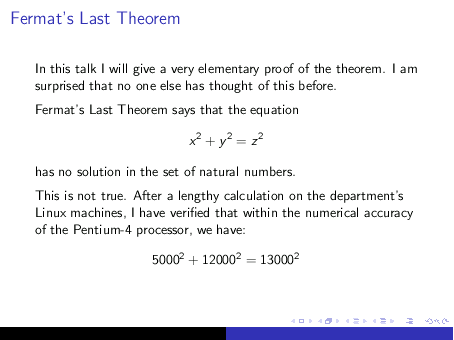# 14  Overlays

Probably the most interesting effect achieved by Beamer is overlays, which gives the appearance of incremental exposure of a given slide.

Achieving the overlay effect in Beamer is quite simple—just insert a \pause command anywhere you want to pause the display.

For instance, to expose an itemized list one-item-at-a-time, do:

\documentclass{beamer}
\usetheme{default}
\begin{document}

\begin{frame}{Outline of the talk}

\begin{itemize}
\item Introduction
\pause
\item Statement of the main theorem
\pause
\item Technical lemmata
\pause
\item Proof of the main theorem
\pause
\item Conclusions
\end{itemize}

\end{frame}

\end{document}


When this file is compiled into the pdf format and displayed with a pdf viewer, such as the Acrobat Reader, the items are exposed one-at-a-time as you page forward through the document in the usual way.

The use of the \pause command is not restricted to itemized lists; you may use it anywhere in a slide. For example:

\documentclass{beamer}
\usetheme{Malmoe}
\begin{document}

\begin{frame}{Fermat's Last Theorem}

In this talk I will give a very elementary proof of the
theorem.  I am surprised that no one else has thought of
this before.
\medskip

\pause

Fermat's Last Theorem says that the equation
$x^2 + y^2 = z^2$
has no solution in the set of natural numbers.
\medskip

\pause

This is not true.  After a lengthy calculation on the
department's Linux machines, I have verified that within
the numerical accuracy of the Pentium-4 processor, we have:
$5000^2 + 12000^2 = 13000^2$

\end{frame}

\end{document}


A pdf viewer will expose the resulting file one paragraph at a time. The fully exposed slide looks like this: